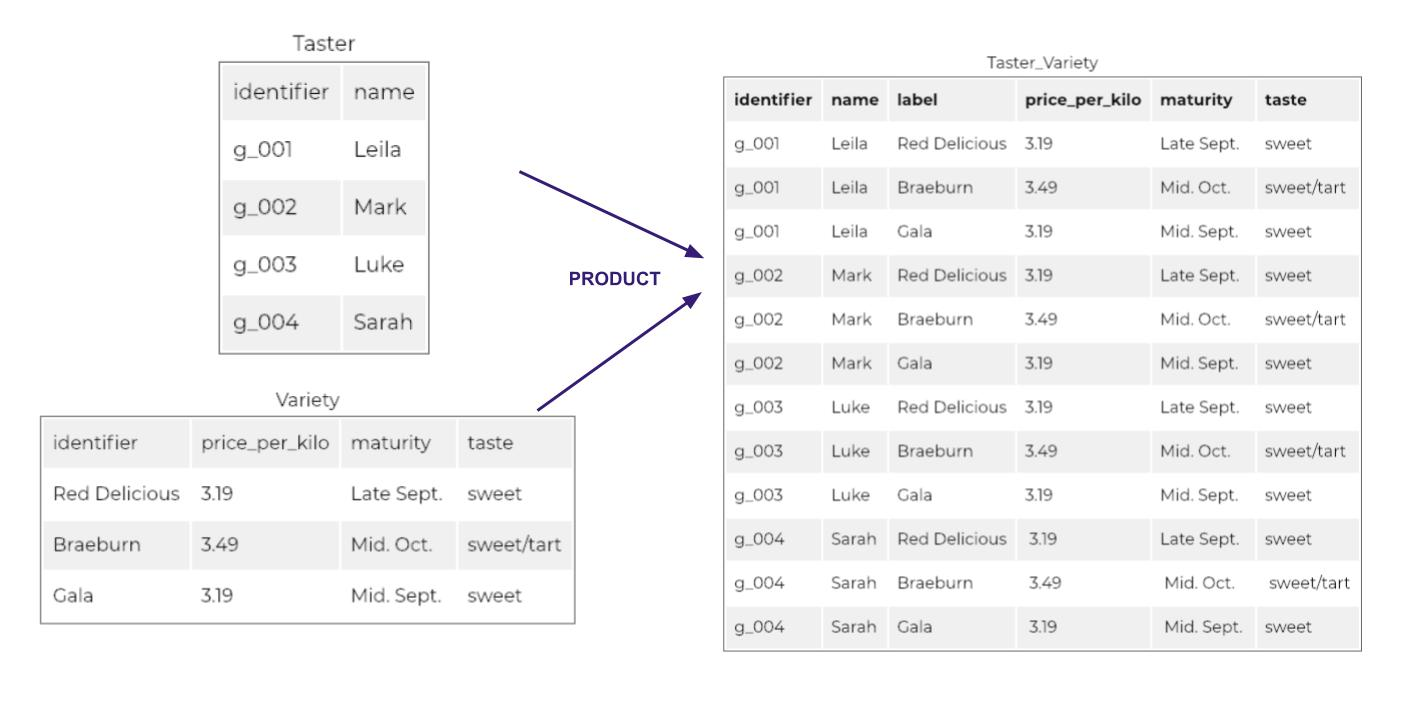Last updated on 4/19/21

## Combine two relations using the cartesian product operation

Log in or subscribe for free to enjoy all this course has to offer!Imagine you are hosting an apple tasting event for 4 people. These 4 people will each be asked to rate 3 different apple varieties.

How many apples do you need to have on hand?

`3 * 4 = 12` You will need a total of 12 apples.

Here we have used the multiplication operation (also known as the "product"), represented by: `*` .

Well, the equivalent in relational algebra is the (Cartesian) product! When you multiply `3 * 4`, you have assumed that each taster will taste each variety. In so doing, you have created all possible combinations of varieties and tasters.

In relational algebra, the Cartesian product of two relations R1 and R2 represents all of the possible combinations of R1 tuples and R2 tuples.

Therefore, if we have a table representing the three varieties of apples, and a table representing our four tasters, the Cartesian product will produce a table:

• that contains the columns of R1 and the columns of R2

• and has rows for all of the apples you will be supplying for tasting.Cartesian Product

So you see that the Cartesian product between a relation with 3 tuples and a relation with 4 tuples results in a relation of 12 tuples!

For the product of relation R1 and relation R2, I suggest the following notation:

`R1 Product R2`

• The Cartesian product between two relations R1 and R2 consists of all the possible combinations of R1 rows and R2 rows.

• The number of rows will be equal to the number of rows of R1 multiplied by the number of rows of R2.